## ML Aggarwal Class 8 Solutions for ICSE Maths Chapter 13 Understanding Quadrilaterals Ex 13.2

Question 1.
In the given figure, ABCD is a parallelogram. Complete each statement along with the definition or property used.
(ii) DC = ………..
(iii) ∠DCB = ………..
(v) ∠DAB = ………..
(vi) OC = ………..
(vii) OB = ………..
(viii) m∠DAB + m∠CDA = ………..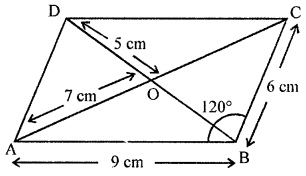Solution:
In paralleloram ABCD
(i) AD = 6 cm (Opposite sides of parallelogram)
(ii) DC = 9 cm (Opposite sides of parallelogram)
(iii) ∠DCB = 60° (∵ ∠DCB + ∠CBA = 180°)
(iv) ∠ADC = ∠ABC = 120°
(v) ∠DAB = ∠DCB = 60°
(vi) OC = AO = 7 cm
(vii) OB = OD = 5 cm
(viii) m∠DAB + m∠CDA = 180°

Question 2.
Consider the following parallelograms. Find the values of x, y, z in each.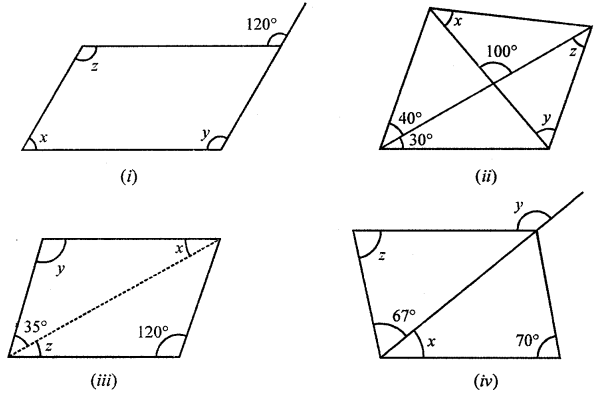Solution:
(i) ABCD is a parallelogram.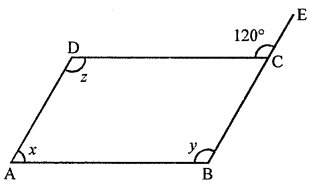Side BC is produced to E
∠DCE = 120°
But ∠DCE + ∠DCB = 180° (Linear pair)
⇒ 120° + ∠DCB = 180°
⇒ ∠DCB = 180° – 120° = 60°
But ∠A = ∠C
⇒ x = 60°
∠DCE = ∠ABC (Corresponding angles)
∴ y = 120°
But z = y (Opposite angle of a ||gm)
⇒ z = 120°
Hence x = 60°, y = 120°, z° = 120°

(ii) In parallelogram ABCD, diagonals bisect each other at O.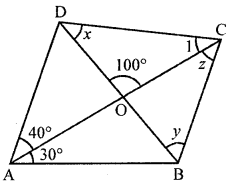∠DAC = 40°, ∠CAB = 30°, ∠DOC = 100°
∠ACB = ∠DAC = 40° (Alternate angles)
∴ z = 40°
∠ACD = ∠CAB (A ltemate angles)
⇒ ∠ACD = 30°
In ∆OCD,
∠DOC + ∠CDO + ∠OCD = 180° (Angles of a triangle)
⇒ 100° + x + 30° = 180°
⇒ x + 130° = 180°
⇒ x = 180°- 130° = 50°
Ext. ∠COD = y + z
100° = y + 40°
⇒ y = 100° – 40° = 60°
∴ x = 50°, y = 60°, z = 40°

(iii) In parallelogram ABCD, AC is its diagonal.
∠B = 120°, ∠DAC = 35°
∠DAB + ∠ABC = 180° (Co-interior angles)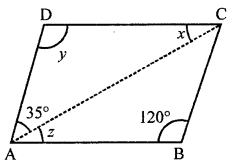35° + z + 120°= 180°
⇒ 155°+ z = 180°
⇒ z = 180° – 155° = 25°
But x = z (Alternate angles)
∴ x = 25°
y = ∠B (Opposite angles of a ||gm)
y = 120°
Hence x = 25°, y = 120°, z = 25°

(iv) In parallelogram ABCD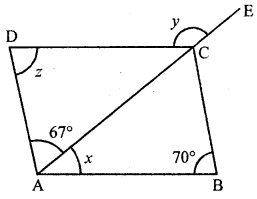∠B = 70°, ∠DAC = 67°
∠D = ∠B (Opposite angles of a ||gm)
⇒ z = 70°
In ∆DAC
Ext. DCE = ∠D + ∠DAC
y = z + 67°
y = 70° + 67°= 137°
and ∠DCA + ∠DCE = 180° (Linear pair)
∠DCA + 137°= 180°
⇒ ∠DCA = 180° – 137° = 43°
But ∠CAB = ∠DCA (Alternate angles)
∴ x = 43°
∴ x = 43°, y = 137°, z = 70°

Question 3.
Two adjacent sides of a parallelogram are in the ratio 5 : 7. If the perimeter of parallelogram is 72 cm, find the length of its sides.
Solution:
In ||gm ABCDAD : AB = 5 : 7
Perimeter of ||gm = 72 cm
⇒ 2(DA + AB) = 72 cm
∴ DA + AB = $$\frac{72}{2}$$ = 36 cm
Let DA = 5x and AB = 7x
5x + 7x = 36
⇒ 12x = 36
⇒ x = $$\frac{36}{12}$$ = 3
∴ AB = 7x = 7 × 3 = 21 cm
AD = 5x = 5 × 3 = 15 cm

Question 4.
The measure of two adjacent angles of a parallelogram are in the ratio 4 : 5. Find the measure of each angle of the parallelogram.
Solution:
In ||gm ABCD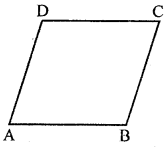∠A : ∠B = 4 : 5
Let ∠A = 4x, ∠B = 5x
But ∠A + ∠B = 180° (Cointerior angle)
∴ 4x + 5x = 180° ⇒ 9x= 180°
⇒ x = $$\frac{180^{\circ}}{9}$$ = 20°
∴ ∠A = 4x = 4 × 20° = 80°
∠B = 5x = 5 × 20° = 100°
But ∠C = ∠A = 80° and ∠D = ∠B = 100°
(Opposite angles of a ||gm are equal)

Question 5.
(i) ∠A + ∠C= 180°?
(ii) AD = BC = 6 cm, AB = 5 cm, DC = 4.5 cm?
(iii) ∠B = 80°, ∠D = 70°?
(iv) ∠B + ∠C= 180°?
Solution:
Quadrilateral ABCD can be a parallelogram of opposite sides
are equal and opposite angles are equal.
∴ ∠A = ∠C and ∠B = ∠D
and AB = DC, AD = BC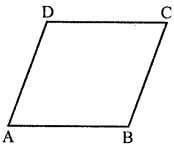(i) ∠A + ∠C = 180°
It may be a parallelogram and may not be.
(ii) ∵ AD = BC = 6 cm, AB = 5 cm, DC = 4.5 cm
∵ AB ≠ DC
(iii) ∠B = 80°, ∠D = 70°
But there are opposite angles and ∠B ≠ ∠D
(iv) ∴ ∠B + ∠C = 180°
It may be or it may not be.

Question 6.
In the following figures HOPE and ROPE are parallelograms. Find the measures of angles x, y and z. State the properties you use to find them.Solution:
(i) In parallelogram HOPE, HO is produced to D∠AOP + ∠POD = 180° (Linear pair)
∴ ∠AOP + 70°= 180°
∠AOP = 180°- 70°= 110°
But ∠AOP = ∠HEP (Opposite angles of a ||gm)
∠HEP = 110°
⇒ x = 110°
∠HPO = ∠EHP (Alternate angles)
∴ y = 40°
In ∆HOP, Ext. ∠POD = y + z
⇒ 70° = y + z
⇒ 70° = 40° + z
⇒ z = 70° – 40° = 30°
∴ x= 110°, y = 40°, z = 30°

(ii) In ||gm ROPE, RO is produced to D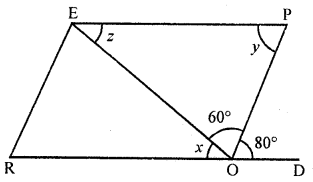∠POD = 80°, ∠EOP = 60°
∠P = ∠POD (Alternate angles)
∴ y = 80°
∠ROE + ∠EOP + ∠POD = 180° (Angles on one side of a line)
x + 60° + 80° = 180° ⇒ x + 140° = 180°
∴ x = 180°- 140° = 40°
z = x (Alternate angles)
∴ z = 40°
Hence, x = 40°, y = 80°, z = 40°

Question 7.
In the given figure TURN and BURN are parallelograms. Find the measures of x and y (lengths are in cm).Solution:
(i) We know that opposite sides of a parallelogram are equal.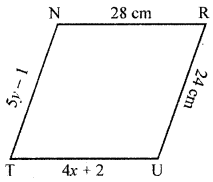∴ TU = RN
4x + 2 = 28 ⇒ 4x = 28 – 2
⇒ 4x = 26
⇒ x = $$\frac{26}{4}$$ = 6.5 cm
and 5y – 1 = 24
⇒ 5y = 24 + 1
⇒ 5y = 25
⇒ y = $$\frac{25}{5}$$ = 5
∴ x = 6.5 cm, y = 5 cm

(ii) We know that the diagonal of a parallelogram bisect each other.∴ BO = OR
⇒ x + y = 20 ………..(i)
and UO = ON
⇒ x + 3 = 18
⇒ x = 18 – 3 = 15 From (i)
15 + y = 20
⇒ y = 20 – 15 = 5
∴ x = 15, y = 5

Question 8.
In the following figure both ABCD and PQRS are parallelograms. Find the value of x.Solution:
Two parallelograms ABCD and PQRS in which
∠A = 120° and ∠R = 50°
∠A + ∠B = 180° (Co-interior angles)
120° + ∠B = 180°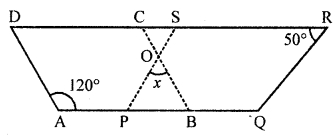⇒ ∠B = 180°- 120° = 60°
∠P = ∠R (Opposite angles of a ||gm)
∠P = 50°
Now in ∆OPB,
∠POB + ∠P + ∠B = 180° (Angles of a triangle)
x + 50° + 60° = 180°
x + 110° = 180° ⇒ x = 180°- 110° = 70°
∴ x = 70°

Question 9.
In the given figure, ABCD, is a parallelogram and diagonals intersect at O. Find :
(ii) ∠ACD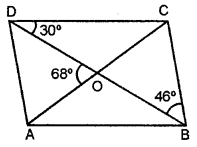Solution:
(i) ∠DBC = ∠BDA = 46° (alternate angles)
In ∆ AOD,
∠CAD = 180°- 114° = 66°
(ii) ∠AOD + ∠COD = 180° (straight angle)
∴ ∠COD= 180°- 68°= 112°
In ∆COD, 112° + 30° + ∠ACD = 180° (∵ ∠ACD = ∠OCD)
∠ACD = 180° – 112° – 30° = 38°

Question 10.
In the given figure, ABCD is a parallelogram. Perpendiculars DN and BP are drawn on diagonal AC. Prove that:
(i) ∆DCN ≅ ∆BAP
(ii) AN = CP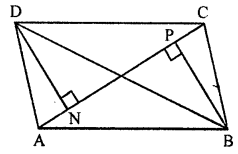Solution:
In the given figure,
ABCD is a parallelogram AC is it’s one diagonal.
BP and DN are perpendiculars on AC.
To prove :
(i) ∆DCN ≅ ∆BAP
(ii) AN = CP
Proof: In ∆DCN and ∆BAP
DC=AB (Opposite sides of a ||gm)
∠N = ∠P (Each 90°)
∠DCN = ∠PAB (Alternate angle)
∴ ∆DCN ≅ ∆BAP (AAS axiom)
∴ NC = AP (c.p.c.t.)
Subtracting NP from both sides.
NC – NP = AP – NP
∴ AN = CP

Question 11.
In the given figure, ABC is a triangle. Through A, B and C lines are drawn parallel to BC, CA and AB respectively, which forms a ∆PQR. Show that
2(AB + BC + CA) = PQ + QR + RP.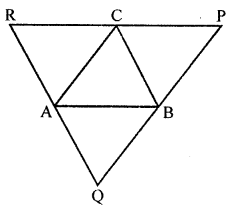Solution:
In the given figure, ABC is a triangle.
Through A, B and C lines are drawn parallel to
BC, CA and AB respectively which forms ∆PQR.To prove:
2(AB + BC + CA) = PQ + QR + RP
∵ BC || PR, AC || RQ
∴ ARBC is a ||gm
∴ AR = CB ….(i)
Similarly ABCP is a ||gm
∴ AP = BC …(ii)
From (i) and (ii),
AR = AP or PR = 2BC …(iii)
Similarly we can prove that
RQ = 2A and PQ = 2AB
Now perimeter of ∆PQR = PQ + QR + RP
= 2AB + 2AC + 2BC
= 2(AB + BC + CA)
Hence PQ + QR + RP = 2(AB + BC + CA)